# Go基础｜第8章：array / slice

• /
• /
• 0
• 2883 次阅读

Synopsis: Go 语言有 3 种数据结构可以让用户管理集合数据：数组（array）、切片（slice）和映射（map），切片类型是在 Go 的数组类型之上所构建的抽象形式（切片会引用其底层数组的一个区间），因此要了解切片，我们必须首先了解数组

# 1. Array

Go 语言的 `数组（array）` 是一个 长度固定 的数据类型，用于存储一段 具有相同的类型 的元素的 连续块。数组存储的类型可以是内置类型，如整型或者字符串，也可以是结构体类型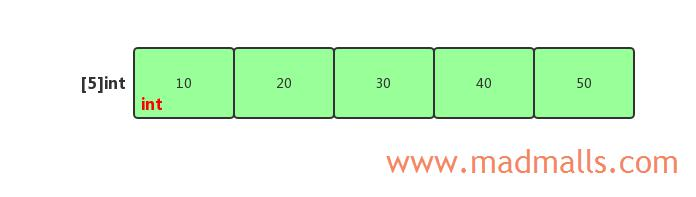## 1.1 声明与初始化

```// 1. 声明一个包含 5 个 int 类型元素的数组
var a int  // [0 0 0 0 0]
```

```// 2. 通过数组字面量（array literal）来声明并初始化数组
a := int{10, 20, 30, 40, 50}

// 3. 使用 ... 代替数组的长度，由初始化的元素个数决定
a := [...]int{10, 20, 30, 40, 50}

// 4. 声明数组，但只初始化其中一部分值，其余的使用对应类型的零值
a := int{2: 20, 4: 40}  // [0 0 20 0 40]
```

## 1.2 访问或修改数组的元素值

```// 1. 下标从 0 开始，获取第 2 个元素的值
fmt.Println(a)

// 2. 修改第 2 个元素的值
a = 8
```

```var a int
b := int{10, 20, 30, 40, 50}
a = b  // 将 b 的值复制给 a，此时 a 和 b 都是 [10 20 30 40 50]
```

## 1.3 迭代数组

```package main

import (
"fmt"
)

func main() {
a := int{10, 20, 30, 40, 50}

for index, value := range a {
fmt.Printf("Index: %d Value: %d\n", index, value)
}
}
```

```Index: 0 Value: 10
Index: 1 Value: 20
Index: 2 Value: 30
Index: 3 Value: 40
Index: 4 Value: 50
```

## 1.4 传递数组

```package main

import (
"fmt"
)

func main() {
// 1. 声明包含 3 个元素的指向 int 的指针数组
var a1 *int

// 2. 声明另一个包含 3 个元素的指向 int 的指针数组
a2 := *int{new(int), new(int), new(int)}
*a2 = 10
*a2 = 20
*a2 = 30

// 3. 将 a2 复制给 a1
a1 = a2

fmt.Println(a1) // [0x414020 0x414024 0x414028]
fmt.Println(a2) // [0x414020 0x414024 0x414028]
}
```

```package arr

// Foo 接受一个指向 100 万个整型值的数组的指针
func Foo(a *int) *int {
return a
}
```

```package arr_test

import (
"testing"
)

func BenchmarkFoo(b *testing.B) {
var a int
for n := 0; n < b.N; n++ {
arr.Foo(&a)  // 传入数组的指针
}
}
```

```D:\MyCode\go-utils\arr>go test -bench=. .
goos: windows
goarch: amd64
BenchmarkFoo-4          1000000000               0.398 ns/op
PASS
```

```package arr

func Foo(a int) int {
return a
}
```

```D:\MyCode\go-utils\arr>go test -bench=. .
goos: windows
goarch: amd64
BenchmarkFoo-4               943           1184240 ns/op
PASS
```

# 2. Slice

`切片（slice）` 是引用类型，它由 3 个字段组成，分别是指向 底层数组 的指针、切片能访问的元素的个数（长度，length）和切片允许增长到的元素个数（容量，capacity）：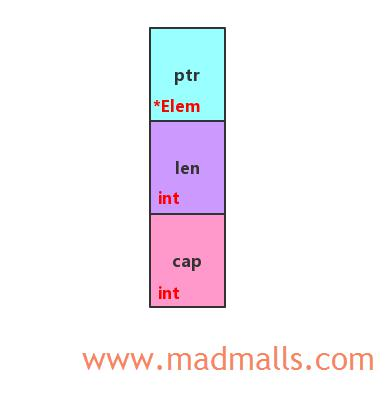## 2.1 声明与初始化

### (1) 数组的切片

```package main

import (
"fmt"
)

func main() {
// 1. 声明并初始化一个数组
a := int{10, 20, 30, 40, 50}
// 2. 创建一个切片，指向底层数组中的第 2 个和第 3 个元素
s := a[1:3]
fmt.Printf("%v len=%d cap=%d\n", s, len(s), cap(s)) // [20 30] len=2 cap=4
}
```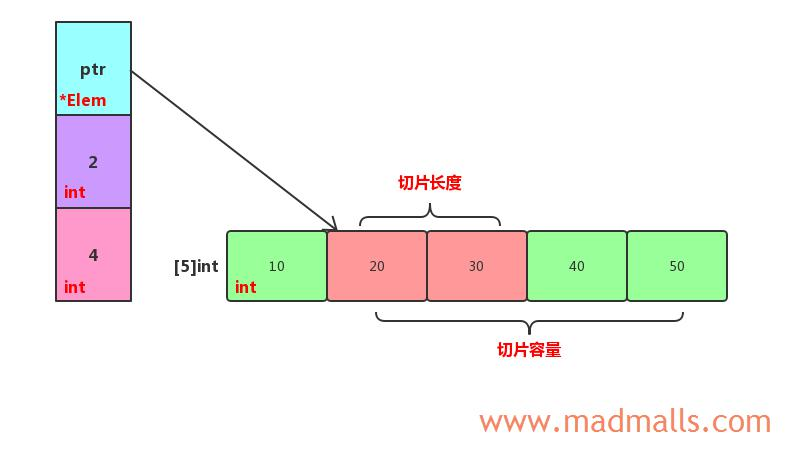• `a[low:high]`： 通过指定起始位置和结束位置来创建切片，它是 `左闭右开` 区间，包括 low 但不包括 high
• 也可以忽略起始位置或结束位置，而使用其默认值。low 的默认值为零，hight 的默认值为数组的长度

```s = a[:2]
fmt.Printf("%v len=%d cap=%d\n", s, len(s), cap(s)) // [10 20] len=2 cap=5
s = a[2:]
fmt.Printf("%v len=%d cap=%d\n", s, len(s), cap(s)) // [30 40 50] len=3 cap=3
s = a[:]
fmt.Printf("%v len=%d cap=%d\n", s, len(s), cap(s)) // [10 20 30 40 50] len=5 cap=5
```

`s := a[1:4]` 时，切片的长度为 3，容量为 4：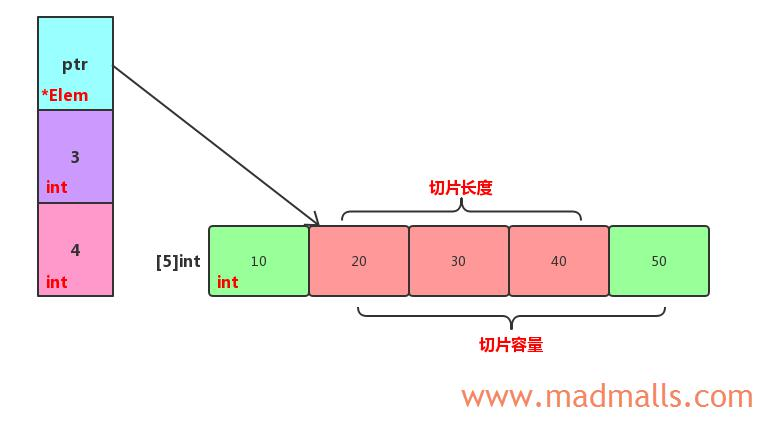### (2) 切片字面量

```s := []int{10, 20, 30, 40, 50}  // 会自动创建底层数组 int{10, 20, 30, 40, 50}
fmt.Printf("%v len=%d cap=%d\n", s, len(s), cap(s)) // [10 20 30 40 50] len=5 cap=5
```

### (3) make() 函数创建切片

```func make([]T, len, cap) []T
```

```s := make([]int, 5)  // 相当于 []int{0, 0, 0, 0, 0}
fmt.Printf("%v len=%d cap=%d\n", s, len(s), cap(s)) // [0 0 0 0 0] len=5 cap=5
```

```s := make([]int, 3, 5)  // 相当于 new(int)[:3]
fmt.Printf("%v len=%d cap=%d\n", s, len(s), cap(s)) // [0 0 0] len=3 cap=5
```

`make([]int, 3, 5)` 表示分配一个具有 5 个 int 类型的数组空间，接着创建一个长度为 3、容量为 5 并指向该数组中前 3 个元素的切片结构

### (4) 切片的切片

• `s[low:high]`： 通过指定起始位置和结束位置来创建切片，它是 `左闭右开` 区间，包括 low 但不包括 high
• 也可以忽略起始位置或结束位置，而使用其默认值。low 和 high 是基于旧切片的索引而不是它的底层数组，所以 low 的默认值为零，high 的默认值为旧切片的长度
• high 不受旧切片的长度限制，但受旧切片的容量限制，意味着你可以用新切片扩展旧切片的长度。如果 high 超过旧切片的容量范围，则会引发 panic 错误；同样地，无法将 low 设置为小于 0 的数来访问底层数组中的前面的元素

```package main

import (
"fmt"
)

func main() {
// 1. 声明并初始化一个切片
s := []int{10, 20, 30, 40, 50}
fmt.Printf("%v len=%d cap=%d\n", s, len(s), cap(s)) // [10 20 30 40 50] len=5 cap=5

// 2. 创建切片的切片
s2 := s[1:3]                                           // low 和 high 是基于切片 [10 20 30 40 50] 的索引
fmt.Printf("%v len=%d cap=%d\n", s2, len(s2), cap(s2)) // [20 30] len=2 cap=4
// s2[:]  // low默认为0、high默认为旧切片的长度，所以 s2[:] 等价于 s2[0:2]， 即 [20 30] len=2 cap=4

// 3. 扩展切片 s2 的长度
s3 := s2[:cap(s2)]                                     // high 最大可设置为旧切片 s2 的容量 4
fmt.Printf("%v len=%d cap=%d\n", s3, len(s3), cap(s3)) // [20 30 40 50] len=4 cap=4

//s4 := s2[:5]  // panic: runtime error: slice bounds out of range [:5] with capacity 4
//s5 := s2[-1:cap(s2)]  // 想访问底层数组第 1 个元素值 10 ? 抱歉会报错：invalid slice index -1 (index must be non-negative)
}
```

### (5) nil 切片和空切片

```package main

import (
"fmt"
)

func main() {
// 1. 创建 nil 切片
var s1 []int
fmt.Printf("%#v len=%d cap=%d\n", s1, len(s1), cap(s1)) // []int(nil) len=0 cap=0

// 2. 通过 slice literal 创建空切片
s2 := []int{}
fmt.Printf("%#v len=%d cap=%d\n", s2, len(s2), cap(s2)) // []int{} len=0 cap=0
// 3. 通过 make() 创建空切片
s3 := make([]int, 0)
fmt.Printf("%#v len=%d cap=%d\n", s3, len(s3), cap(s3)) // []int{} len=0 cap=0
}
```

## 2.2 访问或修改切片的元素值

```package main

import (
"fmt"
)

func main() {
s1 := []int{10, 20, 30, 40, 50}
fmt.Printf("%v len=%d cap=%d\n", s1, len(s1), cap(s1)) // [10 20 30 40 50] len=5 cap=5

s2 := s1[1:3]
fmt.Printf("%v len=%d cap=%d\n", s2, len(s2), cap(s2)) // [20 30] len=2 cap=4

s2 = 8
fmt.Printf("%v len=%d cap=%d\n", s1, len(s1), cap(s1)) // [10 8 30 40 50] len=5 cap=5
fmt.Printf("%v len=%d cap=%d\n", s2, len(s2), cap(s2)) // [8 30] len=2 cap=4
}
```

## 2.3 append() 函数扩容切片

```package main

import (
"fmt"
)

func main() {
a := int{10, 20, 30, 40, 50}
s := a[1:3]
fmt.Printf("%v len=%d cap=%d\n", s, len(s), cap(s)) // [20 30] len=2 cap=4
fmt.Println(a)                                      // [10 20 30 40 50]

s = append(s, 60)
fmt.Printf("%v len=%d cap=%d\n", s, len(s), cap(s)) // [20 30 60] len=3 cap=4
fmt.Println(a)                                      // [10 20 30 60 50]

s = append(s, 70, 80)
fmt.Printf("%v len=%d cap=%d\n", s, len(s), cap(s)) // 切片容量翻倍了，[20 30 60 70 80] len=5 cap=8
fmt.Println(a)                                      // 数组a没变 [10 20 30 60 50]，因为切片指向了新的底层数组
}
```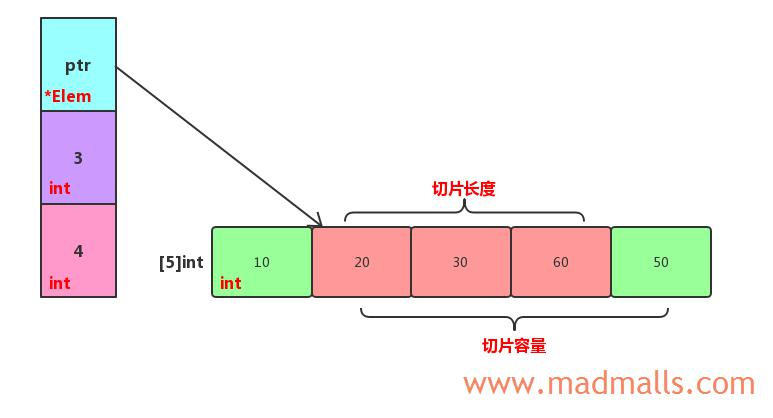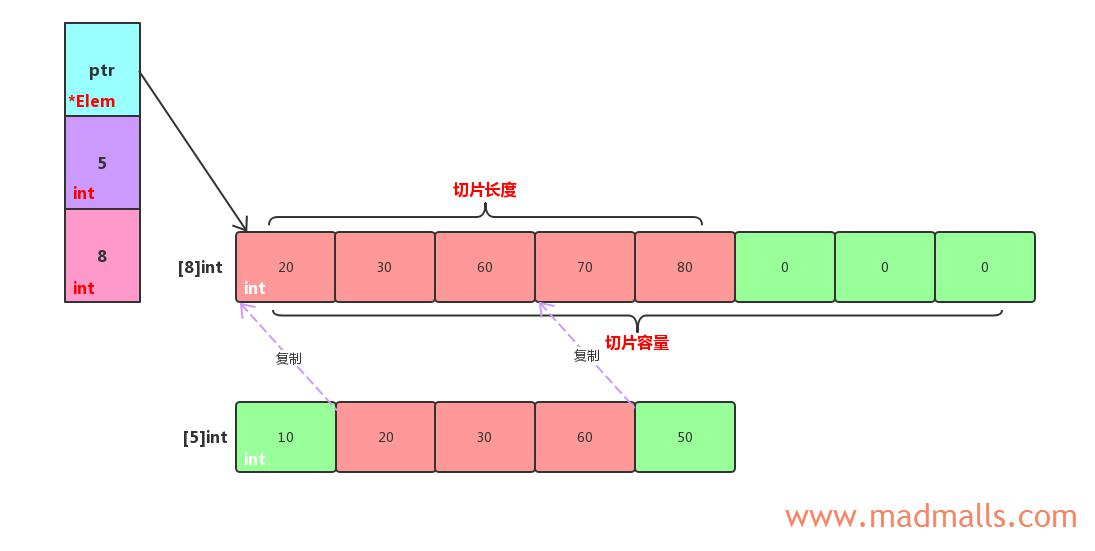```package main

import (
"fmt"
)

func main() {
s1 := []int{10, 20, 30}
s2 := []int{40, 50}
s3 := append(s1, s2...)  // 等价于 append(s1, s2, s2)
fmt.Printf("%v len=%d cap=%d\n", s3, len(s3), cap(s3)) // [10 20 30 40 50] len=5 cap=8
}
```

## 2.4 copy() 函数复制切片

```func copy(dst, src []Type) int
```

```package main

import (
"fmt"
)

func main() {
var s = make([]int, 3)
n := copy(s, []int{10, 20, 30, 40, 50})
fmt.Printf("n=%d\n", n)                             // n = 3
fmt.Printf("%v len=%d cap=%d\n", s, len(s), cap(s)) // [10 20 30] len=3 cap=3

// 相当于给切片 []int{10, 20, 30, 40, 50} 扩充了一倍容量
var s2 = make([]int, 10)
n2 := copy(s2, []int{10, 20, 30, 40, 50})
fmt.Printf("n=%d\n", n2)                               // n = 5
fmt.Printf("%v len=%d cap=%d\n", s2, len(s2), cap(s2)) // [10 20 30 40 50 0 0 0 0 0] len=10 cap=10
}
```

```var digitRegexp = regexp.MustCompile("[0-9]+")

func FindDigits(filename string) []byte {
return digitRegexp.Find(b)
}
```

```func FindDigits(filename string) []byte {
b = digitRegexp.Find(b)
c := make([]byte, len(b))
copy(c, b)
return c
}
```

```func FindDigits(filename string) []byte {
b = digitRegexp.Find(b)
//c := make([]byte, 0)
var c []byte
return append(c, b...)
}
```

## 2.5 切片迭代

```package main

import (
"fmt"
)

func main() {
s := []int{10, 20, 30, 40, 50}
fmt.Printf("%v len=%d cap=%d\n", s, len(s), cap(s)) // [10 20 30 40 50] len=5 cap=5

for index, value := range s {
fmt.Printf("Index: %d Value: %d\n", index, value)
}
}
```

```Index: 0 Value: 10
Index: 1 Value: 20
Index: 2 Value: 30
Index: 3 Value: 40
Index: 4 Value: 50
```

## 2.6 传递切片

```package main

import "fmt"

func Foo(s []int, i int) []int {
s = append(s, i)
return s
}

func main() {
s := make([]int, 0) // empty slice
s = Foo(s, 10)
s = Foo(s, 20)
s = Foo(s, 30)
fmt.Printf("Result: %v", s)
}

/* Output:
D:\MyCode\go-utils>go run transmit_slice.go
Result: [10 20 30]
*/
```

```package main

import "fmt"

func Foo(s *[]int, i int) {
*s = append(*s, i)
}

func main() {
s := make([]int, 0) // empty slice
Foo(&s, 10)
Foo(&s, 20)
Foo(&s, 30)
fmt.Printf("Result: %v", s)
}

/* Output:
D:\MyCode\go-utils>go run transmit_slice.go
Result: [10 20 30]
*/
```

## 2.7 删除切片的元素

### (1) 删除切片的最后一个元素

```package main

import "fmt"

func main() {
s := []int{10, 20, 30, 40, 50}
a := s[len(s)-1]
fmt.Println("Last element:", a) // Last element: 50

// Watch out for memory leaks
s[len(s)-1] = 0 // Erase element (with zero value)
s = s[:len(s)-1]
fmt.Printf("%v len=%d cap=%d\n", s, len(s), cap(s)) // [10 20 30 40] len=4 cap=5
}
```

### (2) 删除切片的中间元素

```package main

import "fmt"

// Remove the element at index i from slice s.
func remove(s []int, i int) []int {
s[i] = s[len(s)-1] // Copy last element to index i
// Watch out for memory leaks
s[len(s)-1] = 0 // Erase element (with zero value)
s = s[:len(s)-1]
return s
}

func main() {
s := []int{10, 20, 30, 40, 50}

s = remove(s, 2)
fmt.Printf("%v len=%d cap=%d\n", s, len(s), cap(s)) // [10 20 50 40] len=4 cap=5
}
```

```package main

import "fmt"

// Remove the element at index i from slice s.
func remove(s []int, i int) []int {
copy(s[i:], s[i+1:]) // Shift s[i+1:] left one index
// Watch out for memory leaks
s[len(s)-1] = 0 // Erase element (with zero value)
s = s[:len(s)-1]
return s
}

func main() {
s := []int{10, 20, 30, 40, 50}

s = remove(s, 2)
fmt.Printf("%v len=%d cap=%d\n", s, len(s), cap(s)) // [10 20 40 50] len=4 cap=5
}
```

## 2.8 清空切片

### (1) 会释放内存

```package main

import "fmt"

func main() {
s := []int{10, 20, 30, 40, 50}
fmt.Printf("%v len=%d cap=%d\n", s, len(s), cap(s)) // [10 20 30 40 50] len=5 cap=5

s = nil
fmt.Printf("%v len=%d cap=%d\n", s, len(s), cap(s)) // [] len=0 cap=0
}
```

### (2) 会保持内存

```package main

import "fmt"

func main() {
s := []int{10, 20, 30, 40, 50}
fmt.Printf("%v len=%d cap=%d\n", s, len(s), cap(s)) // [10 20 30 40 50] len=5 cap=5

s = s[:0]
fmt.Printf("%v len=%d cap=%d\n", s, len(s), cap(s)) // [] len=0 cap=5

s = s[:3]
fmt.Printf("%v len=%d cap=%d\n", s, len(s), cap(s)) // [10 20 30] len=3 cap=5
}
```

## 作者